# Variables

In Proto.io, “variables” is a mechanism with which you can store and reuse user defined values (numbers, text etc). For example you can store the contents of an input box in a variable, and later set the text of a label using the value of that variable.

Variables are global within your project, meaning that you can use them throughout your prototype. Variables are managed within the Editor under “Variables Manager”.

Variables' names have to be unique, using alphanumeric characters and should ideally reflect the variable’s purpose. Variables can only hold one value at a time and you can optionally give them initial values upon definition. Variable types can be numeric, text, or boolean.

Variables are used whenever a value must be stored so that it can affect the User Interface in some way at some point. More specifically, once your variable is created, you can assign a value either upon creation (via Variable Manager), or at any other point in your prototype (through the Interaction Modal -> Set Variable) and then be able to read that value by referring to that variable. The ‘Evaluate’ option (seen in the image below) is needed if the variable’s value is given through an expression (see Advanced section below), in order to evaluate it before it’s used. Manipulating variable values dynamically (throughout the course of the prototype’s play) advances your prototype’s interaction by making it react to events based on conditional logic in a simple and straightforward way. When you copy screens/containers between projects, then the variables that are used are copied as well.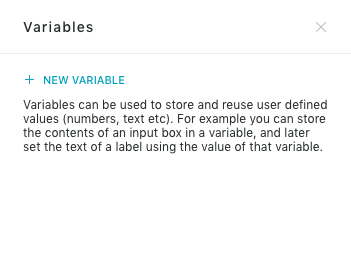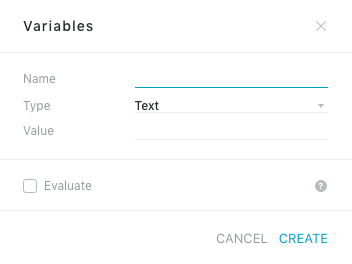### Setting the value of a variable

After you create your variables you can give them values upon different events such as the user performing some sort of interaction e.g. clicking on a button. To do that, you select your item containing the interaction (e.g. your button) and in the Interaction Modal, you select “Set variable…” as the preferred action. You then choose the variable to which you want to assign a value to. The Callback option in this Modal lets you define another interaction that will be executed after the main one is completed.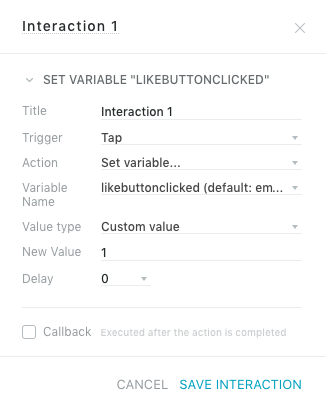Screenshot of the Properties Inspector (Interactions tab), where a variable ‘likebuttonclicked’ is given the value “1” when the user clicks on the button.

Then you have the following options:

 Value Type Explanation Custom Value You can specify a value or a mathematical expression that will be evaluated. You can also refer to other variables using their names in curly braces, e.g. {slider_position}, {checkbox_value}. Set value from item property You can choose your variable’s value to be taken from a selected UI element’s property. Callback value from event You can choose your variable’s value to be dependent on the trigger of the specific UI element’s interaction. For example, you can choose your variable’s value to be the new value set by the user for the particular UI element.

Variables can also be read so as to change a UI element’s property. See image below.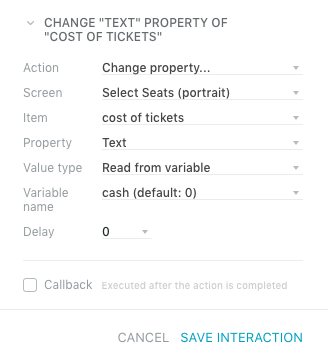### Examples

A common example of variable use is when your prototype accepts user input and is designed to use that in some way. That input can be stored in a variable and used throughout your project by referring to that variable. See an example below where the text entered in a text field is captured upon tapping the button and affects a text area in a different screen.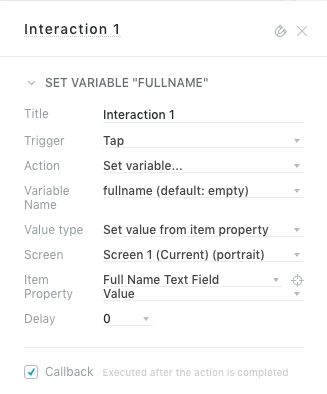Setting variable ‘fullname’ to the value of text field ‘Full Name Text Field’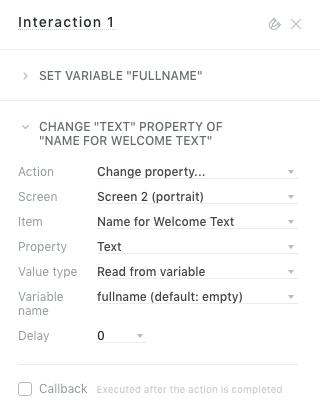Using the ‘fullname’ variable value to replace a text property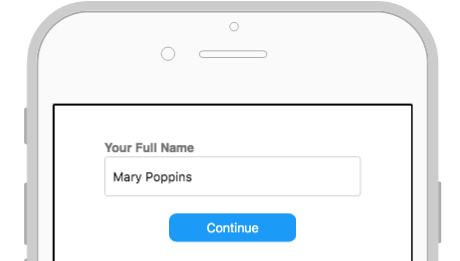The User Interface showing the text input field and the button on which the interactions are attached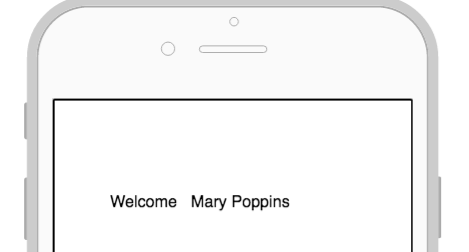Example output on screen

Another typical example is when you want your prototype to behave in a particular way, based on a UI element’s property value or change of value. For example, when you want to change a text label somewhere on the interface, based on the month a user selected from a month picker UI element. See images below.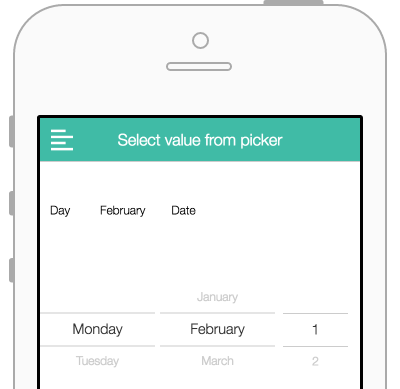The User Interface showing the Month Picker on which the interaction is built and the ‘Month text’ shown above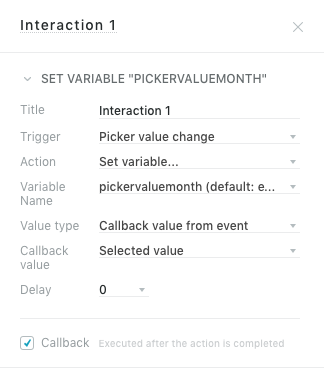Setting variable ‘pickervaluemonth’ to what the user selects from the Month Picker UI element.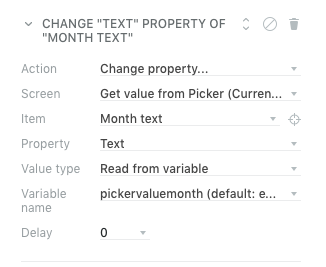Reading the ‘pickervaluemonth’ variable value and changing the ‘Month text’ text property accordingly

For more advanced use of variables you can refer to the table below with more complex expressions to achieve certain effects. These are to be entered in the ‘New Value’ field, if ‘Custom Value’ is selected for Value Type. Note that the Javascript Math functions can also be used.

 Custom Value Variable Type Expected result {var1} {var2} text Get a string of the two variables separated by a space {var1} and {var2} text Get a string of the two variables separated by an “and” {var1} + {var2} numeric Add variables {var1} – {var2} numeric Subtract variables {var1} * {var2} numeric Multiply variables {var1} / {var2} numeric Divide variables Math.max({var1},{var2}) numeric Get the maximum of the two variables Math.round({var1}+{var2}) numeric Get the rounded sum of the two variables ({var1}+{var2}).toFixed(2) numeric Get the rounded sum of the two variables with 2 decimal points Math.cos({var1}) numeric Calculates the cosine of var1. This will be a value from -1.0 to 1.0. Math.sin({var1}) numeric Calculates the sine of var1. This will be a value from -1.0 to 1.0. Math.tan({var1}) numeric Calculates the tangent of var1. Math.abs({var1}) numeric Returns the absolute value of the number stored in var1. Math.ceil({var1}) numeric Returns the smallest integer greater than or equal to the number stored in var1. Math.floor({var1}) numeric Returns the largest integer less than or equal to the number stored in var1. Math.random() numeric Returns a pseudo-random number between 0 and 1. Math.trunc({var1}) numeric Returns the integral part of the number stored in var1, removing any fractional digits. "{var1}".length numeric Returns the length of a variable "{var1}".toString().replace(/\B(?=(\d{3})+(?!\d))/g, ","); numeric Adds a comma between every 3 digits "{var1}".replace(".", ",") text Replaces a . (period) with a , (comma) {var1}1 text Will add the character 1 next to the variable’s value (keypad example) {var1}+1 numeric Will result an increment on the variable’s value by 1 ({var1} == 0 ) ? 1 : 0 numeric IF var1 is equal to 0 THEN value is 1 ELSE value is 0 ({var1} < 0 ) ? 1 : 0 numeric IF var1 is less than 0 THEN value is 1 ELSE value is 0 ({var1} <= 0 ) ? 1 : 0 numeric IF var1 is less than or equal to 0 THEN value is 1 ELSE value is 0 ({var1} > 0 ) ? 1 : 0 numeric IF var1 is greater to 0 THEN value is 1 ELSE value is 0 ({var1} >= 0 ) ? 1 : 0 numeric IF var1 is greater or equal to 0 THEN value is 1 ELSE value is 0 ({var1} != 0 ) ? 1 : 0 numeric IF var1 is not equal to 0 THEN value is 1 ELSE value is 0 ({var1} < 50) ? -1 : (({var1} > 50 ) ? 1 : 50) numeric IF var1 is less than 50 THEN value is -1 ELSE IF var1 is greater than 50 value is 1 ELSE value is 50 ("{var1}" == "John" ) ? 1 : 0 numeric IF var1 is equal to text John THEN value is 1 ELSE value is 0 "{var1}" == "email1" ? "State 2" : ("{var1}" == "email2" ? "State 3" : "State 1") text Set a variable that can be later used to change the State of a Container ({var1} == 1 && {var2} == 1) ? 1 : 0 numeric IF var1 is 1 AND var2 is 1 THEN value is 1 ELSE value is 0 new Date().toLocaleDateString('en-GB') text Today’s date in format dd/mm/yyyy new Date().toLocaleDateString('en-US') text Today’s date in format mm/dd/yyyy new Date(new Date().getFullYear(), new Date().getMonth(), new Date().getDate() + 1).toLocaleDateString('en-US') text Tomorrow’s date in format mm/dd/yyyy new Date(new Date().getFullYear(), new Date().getMonth(), new Date().getDate() + 10).toLocaleDateString('en-US') text Date in Ten days from today in format mm/dd/yyyy new Date().toLocaleTimeString('en-GB').replace(/(.*)\D\d+/, '\$1') text This will return the current time ["Sunday","Monday","Tuesday","Wednesday","Thursday","Friday","Saturday"] [new Date().getDay()] text This will return the current day ['January', 'February', 'March', 'April', 'May', 'June', 'July', 'August', 'September', 'October', 'November', 'December'][new Date().getMonth()] text This will return the current month ("{var1}".substr(0,1)).toUpperCase() text Get the first letter of a string Capitalised "{inputvalue}".match(/[^|]*/) text This will return the characters before the "|" "{inputvalue}".match(/\|(.*)/) text This will return the characters after the "|"

### Example

You have two text input fields on your User Interface, one for Firstname and one for Lastname. The data the user enters is kept in two dedicated variables (‘first_name’ and ‘last_name’ respectively). Then these are brought together to create variable ‘full_name’ in order to later customize a text message.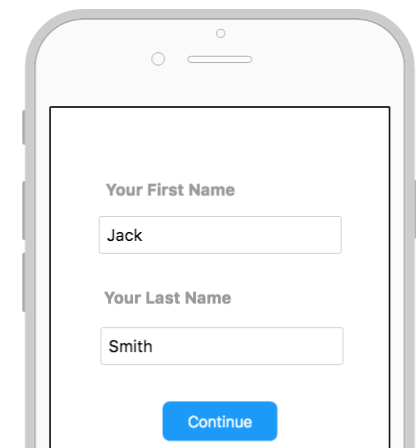UI with two text input fields and a button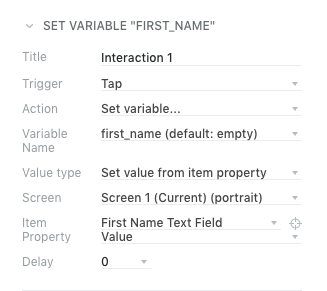Setting variable ‘first_name’ to what the user entered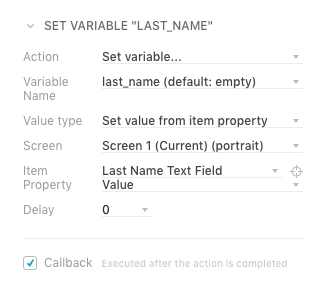Setting variable ‘last_name’ to what the user entered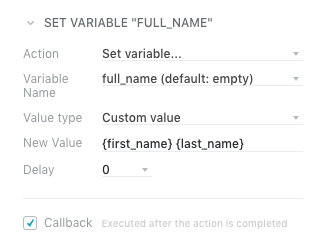Setting a new variable ‘full_name’ to value that results from merging ‘first_name’ and ‘last_name’ separated by SPACE.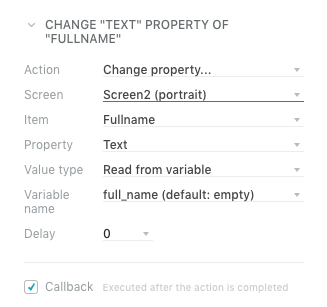Changing a text property according to ‘full_name’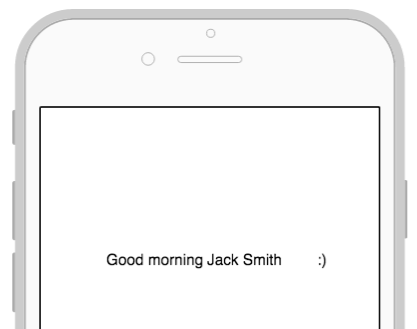Example output

For more information, you can check out our "Introduction to Variables" video tutorial and our "Using Variables for Advanced Prototyping" webinar.

You can also check out the ‘Variables Examples’ project in Spaces:

https://spaces.proto.io/project/58DD496F-D061-F782-11B1-13EDF39601FE/Variables-Examples/

Have more questions? Submit a request